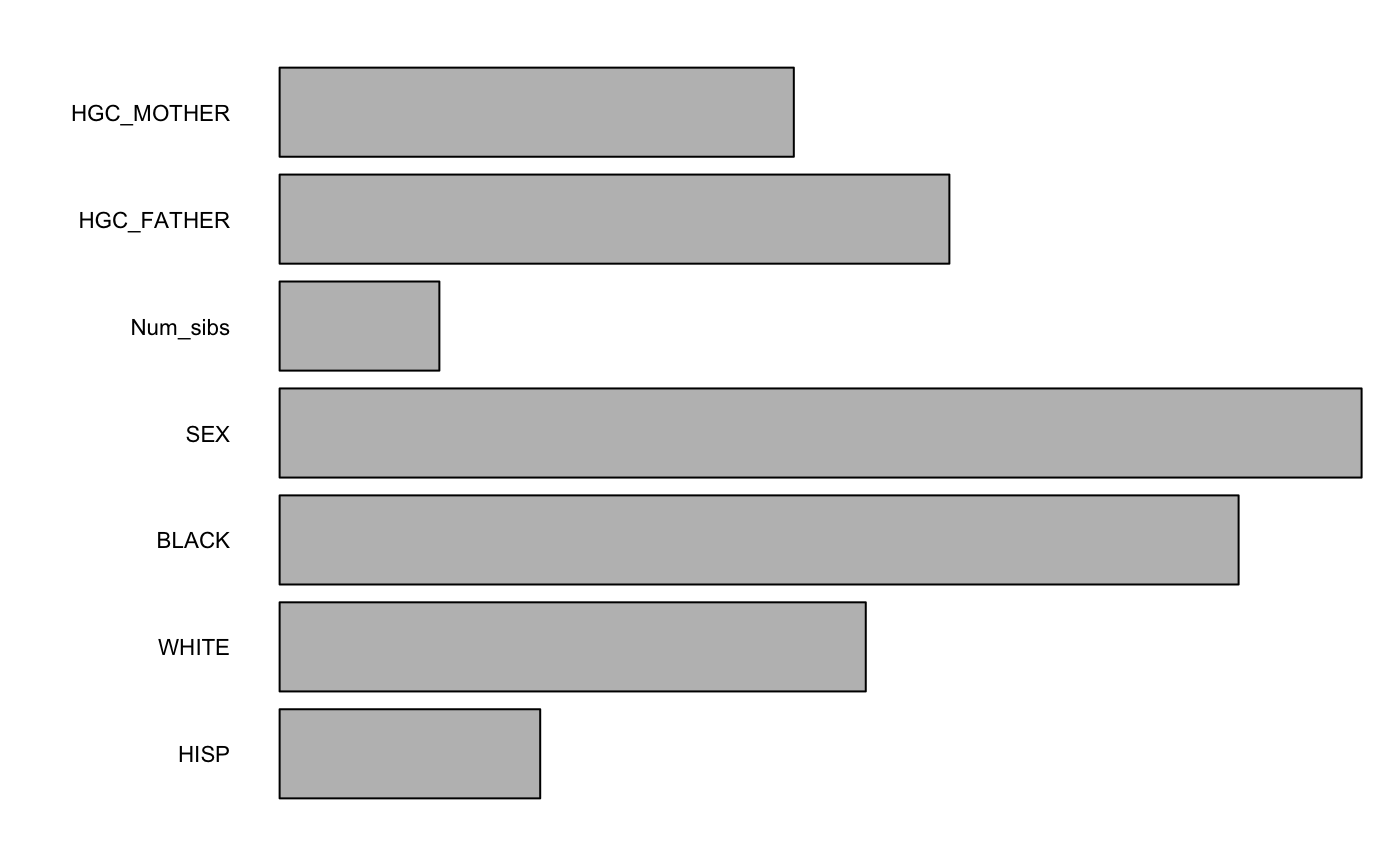Returns the random forest variable importance based on the permutation accuracy measure, which is calculated as the difference in mean squared error between the original data and from randomly permuting the values of a variable.

varImpY(forest, method = "oob")

Arguments

forest a random forest, generated from splineForest() the method to be used. This must be one of "oob" (out of bag), "all", "itb" (in the bag).

Value

A matrix storing variable importance metrics. The rows correspond to split variables. The columns are different methods of measuring importance. The first column is the absolute importance (mean difference in performance between permuted and unpermuted datasets). The second column measures the mean percent difference in performance. The third column standardizes the differences by dividing them by their standard deviation.

Details

The "method" parameter deals with the way in which forest performance should be measured. Since variable importance is based on a change in performance, the "method" parameter is necessary for a variable importance measure. The choices are "oob" (out of bag), "all", or "itb" (in the bag).

Examples

importanceMatrix <- varImpY(forest, method="oob")
#>  "Working on tree: " #>  1 #>  2 #>  3 #>  4 #>  5 #>  6 #>  7 #>  8 #>  9 #>  10
plotImp(importanceMatrix[,3])